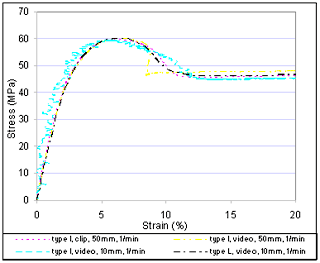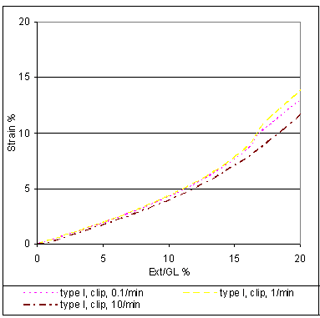A Novel Technique to Measure Tensile Properties of Plastics at High Strain Rates

Luke Perkins Cornell University, Ithaca NY, Hubert Lobo DatapointLabs, Ithaca NY

Abstract

High strain-rate properties have many applications in the simulation of automotive crash and product drop testing.
These properties are difficult to measure. These difficulties result from inaccuracies in extensometry at high strain
rates due to extensometer slippage and background noise due to the sudden increase in stress at the start of the
test. To eliminate these inaccuracies we use an inferential technique that correlates strain to extension at low
strain rates and show that this can be extended to measure strain at higher strain rates

Introduction

High strain rate tests have many applications in industry but it is often difficult to obtain accurate data at high
strain rates. These tests are often run on a Universal Testing Machine (UTM) with an extensometer attached
to a small specimen to measure strain. The extensometer most often is the cause of inaccurate strain readings
when testing at high strain rates. These errors can result from slipping, inertial effects from the mass of the
extensometer, and also from background noise at the start of the test. Non-contact devices such as laser
or video extensometers have been used but these are also limited by vibration and noise.

In this paper, it is proposed that strain should be measured by crosshead extension rather than by an extenso-
meter at high strain rates to avoid these errors. The crosshead extension of a UTM can be correlated to the strain
of a specimen at low strain rates using an extensometer. If this relationship is independent of strain rate, then the
relationship between crosshead displacement and strain can be used to find strain values for tests run at a
higher strain rates.

The tests were run to verify that there is indeed a unique relationship between crosshead extension and strain.
The material used was a polycarbonate.

 Methods Two series of tests were conducted to determine if crosshead extension can be used to calculate strain. All tests were based upon ASTM D638. The first series of tests compared the results of four different testing variations all conducted at the same strain rate of 1/min. The purpose of this test was to verify that stress, strain, and extension data are independent of test specimen type and method of extensometry, and that the test methods accurately represent the material properties. Table 1 provides details. ASTM D638 Type I specimens are most commonly used for conventional tensile testing; the ASTM D1822 Type L specimens are preferred for high strain work because their reduced cross sectional area allows higher strain rates to be reached using the same speed that would be applied to a type I specimen at a lower strain rate. These tests were designed to show that type L specimens behave in the same manner as type I specimens and that video extensometers give the same results as clip on extensometers. One variable was changed at a time so that any difference in the results could be attributed to that variable. The video extensometer was set to twenty-five acquisitions per second. The results of these four tests are plotted on Figures 1 and 2. Figure 1 shows nominal strain (crosshead displacement divided by gauge length) versus measured strain. Figure 2 shows stress versus measured engineering strain. Measured strain (ε) is calculated by dividing the change of gauge length (ΔL) by the initial gauge length (L). ε = ΔL / L (1) If it is necessary to establish a relationship between extension and strain for two different types of test specimens then the different gauge lengths of the specimens need to be taken into account. If both specimen types are stretched an equal distance the strain calculated for the type I specimen will be five times smaller than the strain calculated for the type L specimen because the gauge region is five times larger. In order to compare the strains of the two different specimens a scaling factor of five is needed. The strains from the type I specimens must be multiplied by five, thus changing the effective gauge region of the samples from 50mm to 10mm. Once the effective gauge region has been normalized the type L and type I specimens can be compared directly. The second series of tests were conducted to determine how strain correlates to crosshead displacement at varying strain rates. These tests were conducted using only type I specimens. Type I specimens were tested at 0.1/min, 1/min, and 10/min which covers the range of strain rates that were possible on UTM1. Nominal strain versus measured strain was plotted to see if the curves at different strain rates would overlap therefore showing that the relationship between crosshead extension and strain was independent of strain rate. Finally a test was run on UTM2 using type L test specimens without and extensometer. Results Figures 1 and 2 show that for all four testing methods the relationship between nominal strain and engineering strain is the same. The relationship holds well up to the yield point; after yield the graphs do not correlate as well. Explanations for this occurrence include the video extensometer losing sight of the gauge region, the clip extensometer slipping, or variable post yield behavior. The second series of tests takes the relationship between extension and strain one step further to show that strain can be calculated from crosshead displacement at any strain rate. Since the different testing methods all produced the same result at one strain rate only one of the testing methods was needed for testing at multiple strain rates. The type I specimens were tested at 0.1/min, 1/min, and 10/min and the results were plotted. Figure 3 shows nominal strain versus strain at different strain rates. For each of the strain rates the curves line up showing that strain and extensometer extension relate in the same way regardless of strain rate. Figure 4 shows the Stress versus Strain curves at varying strain rates. These plots show that for this material the maximum stress occurs at the same strain value every time but that the yield stress increases as strain rate increases. To obtain high strain rate properties specimens were tested first on the UTM1 using clip-on extensometer to find how strain relates to crosshead displacement. Using the data from that test a polynomial was fit to relate strain to crosshead displacement. Using the polynomial fit curve, strain measurements were obtained using crosshead displacement data from the high speed experiment. Results are shown in Figure 4, graph labeled polynomial. Figure 5, an Eyring plot, shows that as strain rate increases the maximum value of the stress also increases in a linear fashion. Conclusions These tests show that an extensometer can be used to establish the relationship between crosshead extension and strain. An extensometer must be used to find the strain values that correlate to a given crosshead extension. Once this correlation is made an extensometer is no longer necessary provided that the relationship between crosshead displacement and strain is independent of strain rate. For the polycarbonate tested, the relationship between strain and crosshead extension is independent of specimen type, extensometry and strain rate. As a result tests of type I specimen and type L specimen can be compared directly. Tests can be run using both specimen types for instance, a type I specimen can be used to find the relationship between crosshead extension and strain and then further testing can be conducted using a type L specimen without an extensometer. After peak stress, (yield point) is achieved the correlation between nominal strain and measured strain is not as good. This is because after the yield point the measured strain values for the different testing methods vary greatly. These variations result from error in extensometry and may be caused by extensometer slip or by deterioration of the markings used for the video extensometer. Only fair correlation could be obtained in the post yield region. A variable that can affect the relationship between crosshead extension and strain is temperature. These tests were conducted at constant room temperature, 23°C; therefore they do not demonstrate any correlation between crosshead extension and strain at variable temperatures. Further, it needs to be shown that this methodology applies to plastics other than polycarbonate. Equipment UTM1: Instron 5566 servo-mechanical Universal testing machine. UTM2: Instron 8872 servo-hydraulic universal testing machine Clip Extensometer: Instron 2630-100, 2in linear clip-on extensometer Video Extensometer: Hitachi KP-M2U Acknowledgements We wish to thank Professor Alan Zehnder, Cornell University for his advice and expertise. Thank you to Mr. Brian Croop, DatapointLabs for consulting with us during the testing phase of our project. Thank you to Mr. Brian Lussier, DatapointLabs for running many of the tests necessary to complete this study. References . 1. Lobo, H., Lorenzo, J., “High Speed Stress-Strain Material Properties As Inputs For The Simulation Of Impact Situations,” IBEC, Stuttgart, Germany (1997). 2.Grimson, A., Sinopoli, M., Lobo, H., Fedewa, B., “Evaluation of High Strain Rate Test Methods of Thermoplastic Polyolefins Used for Automotive Interior Structural Analyses,” SPE TPO Conference, Detroit, (2003). 3. Croop, B., “Developing Material Model Parameters for Impact Simulation of Plastics and Foams,” ABAQUS User’s Conference, Boston (2004).Table 1 The table outlines the different testing methods used in this study.Figure 1 Data from UTM1 run at a constant strain rate. The graph shows all methods give the same results.Figure 2 Data from UTM1 at constant strain rate. Shows that each method gives the same results.Figure 3 Data from UTM1 shows that the relationship between strain and nominal strain is rate independent.Figure 4 Data from UTM1 and UTM2 showing how stress and strain vary at different strain rates.Figure 5 Eyring plot to show that yield stress increases as strain rate increases.